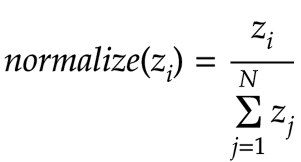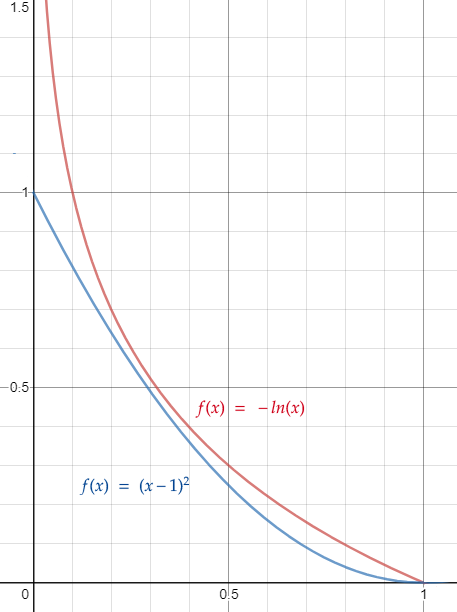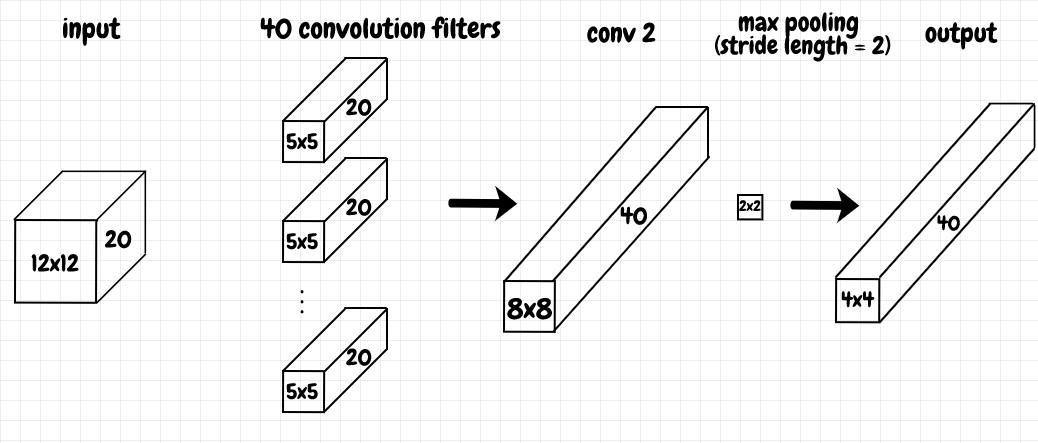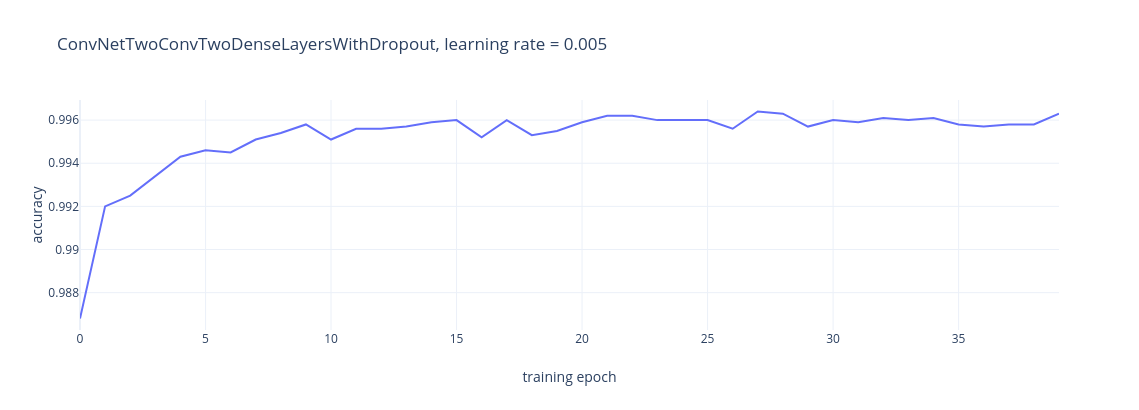09 Sep 2019

# PyTorch Image Recognition with Convolutional Networks

### PyTorch (3 Part Series)

3. PyTorch Image Recognition with Convolutional Networks

In the last article, we implemented a simple dense network to recognize MNIST images with PyTorch. In this article, we’ll stay with the MNIST recognition task, but this time we’ll use convolutional networks, as described in chapter 6 of Michael Nielsen’s book, Neural Networks and Deep Learning. For some additional background about convolutional networks, you can also check out my article Convolutional Neural Networks: An Intuitive Primer.

We’ll compare our PyTorch implementations to Michael’s results using code written with the (now defunct) Theano library. You can also take a look at the underlying framework code he developed on top of Theano. PyTorch seems to be more of a “batteries included” solution compared to Theano, so it makes implementing these networks much simpler. The dense network from the previous article had an accuracy close to 98%. Our ultimate goal for our convolutional network will be to match the 99.6% accuracy that Michael achieves.

The code for this project is available on github, mainly in pytorch_mnist_convnet.py.

## Simple Convolutional Network

The first convolutional network design that Michael presents is `basic_conv`. Our PyTorch implementation is shown below (pytorch_mnist_convnet.py):

``````class ConvNetSimple(nn.Module):
def __init__(self):
super().__init__()
self.conv1 = nn.Conv2d(in_channels=1, out_channels=20, kernel_size=5)
self.fc1 = nn.Linear(12*12*20, 100)
self.out = nn.Linear(100, OUTPUT_SIZE)

def forward(self, x):
x = self.conv1(x)
x = torch.sigmoid(x)
x = torch.max_pool2d(x, kernel_size=2, stride=2)

x = x.view(-1, 12*12*20)
x = self.fc1(x)
x = torch.sigmoid(x)

x = self.out(x)
return x
``````

In this network, we have 3 layers (not counting the input layer). The image data is sent to a convolutional layer with a 5 × 5 kernel, 1 input channel, and 20 output channels. The output from this convolutional layer is fed into a dense (aka fully connected) layer of 100 neurons. This dense layer, in turn, feeds into the output layer, which is another dense layer consisting of 10 neurons, each corresponding to one of our possible digits from 0 to 9.

The `forward` method is called when we run input through the network. We use sigmoid activation functions for each of our layers, except for the output layer (we’ll look at this in more detail in the next few sections). We also compress the output from our convolutional layer in half by applying 2 × 2 max pooling to it, with a stride length of 2. The diagram below shows the structure of this network:In the previous article, we saw that the data returned by the loader has dimensions `torch.Size([10, 1, 28, 28])`. This means there are 10 images per batch, and each image is represented as a 1 × 28 × 28 grid. The 1 means there is a single input channel (the data is in greyscale). The diagram below shows in more detail how the input is processed through the convolutional layer:In SciPy, `convolve2d` does just what is says: It convolves two 2-d matrices together. The behaviour of `torch.nn.Conv2d` is more complicated. The line of code that creates the convolutional layer, `self.conv1 = nn.Conv2d(in_channels=1, out_channels=20, kernel_size=5)`, has a number of parts to it:

• `kernel_size` tells us the 2-d structure of the filter to apply to the input. We can supply this as tuple if we want it to be a rectangle, but if we specify it as a scalar, as we do here, then that value is used for both the height and width, a 5 × 5 square in this case.
• `in_channels` extends the kernel into the 3rd dimension, depth-wise. These three parameters, the height and width of the kernel, and the depth as specified by the number of input channels, define a 3-d matrix. We can convolve the 3-d input with this 3-d filter. The result is a 24 × 24 2-d matrix. This 2-d matrix is a feature map. Each neuron in this feature map identifies the same 5 × 5 feature somewhere in the receptive field of the input.
• `out_channels` tells us how many filters to use - in other words, how many feature maps we want for the convolutional layer. The 2-d outputs from the convolution of the input with each filter are stacked on top of one another.

Even though I think of this as a 3-d operation (especially when there is more than one input channel), I guess it’s called `Conv2d` in PyTorch to indicate that each channel has a 2-dimensional shape (`Conv3d` is used when each channel has 3 dimensions). I go into more detail about forward and back propagation through convolutional layers in Convolutional Neural Networks: An Intuitive Primer.

Conceptually, each filter produces a feature map, which represents a feature that we’re looking for in the receptive field of the input data. In this case, that means the network learns 20 distinct 5 × 5 features. During forward propagation, `max_pool2d` compresses each feature. It’s applied to each channel, turning each 24 × 24 feature map into a 12 × 12 matrix for each channel. The result is a 3-d matrix with the same depth (20 channels in this case).

Note, as shown below, that `Conv2d` technically performs a cross-correlation rather than a true convolution operation (`Conv2d` calls `conv2d` internally):

``````>>> import torch
>>> from torch import nn
>>> from scipy import signal
>>> values = torch.tensor([[[[1,2,3],[4,5,6],[7,8,9]]]])
>>> f = torch.tensor([[[[10,20],[30,40]]]])
>>> nn.functional.conv2d(values, f)
tensor([[[[370, 470],
[670, 770]]]])
>>> signal.correlate2d(values[0,0], f[0,0], mode="valid")
array([[370, 470],
[670, 770]], dtype=int64)
``````

## Softmax

We want the output to indicate which digit the image corresponds to. In other words, we want the output for the correct prediction to be as close to 1 as possible, and for the rest of the outputs to be as close to 0 as possible.

First, we will normalize our outputs so that they add up to 1, thus turning our ouput into a probability distribution. The simple way to normalize our outputs would be just to divide each output by the sum of all of the outputs (N is the number of outputs):We will use a function called softmax instead. With softmax, we adjust the above formula by applying the exponential function to each output:Why should we do this? I don’t think Michael compares softmax with the simple linear normalization shown earlier. One benefit is that, with softmax, the highest output value will get an exponentially greater proportion of the total. This encourages our network to more sharply favour the highest output over all of the others. This approach also has the advantage that any negative outputs will be automatically converted to positive values - since the exponential function returns a positive value for any input (it approaches 0 as x goes to negative infinity).

You may also want to see what Michael has to say about softmax in Neural Networks and Deep Learning, as he goes into some interesting additional discussion of its properties.

## Negative Log Likelihood Loss

Once we have the output transformed with softmax, we need to compute the loss. For this, we’ll use the negative log likelihood loss function. For the target value, where we want the probability to be close to 1, the loss is f(x) = -ln(x), where x is the network’s output for the desired prediction. Why should we use the negative log instead of our old friend, the quadratic cost function? I found it helpful to compare negative log against the quadratic cost, f(x) = (x - 1)2:We can see that the cost goes down to 0 for both functions as the output approaches 1, which is what we want. The advantage of the log likelihood over quadratic cost is that the cost for log likelihood rises much faster as the output moves away from 1 and toward 0. That means the gradients we compute get much higher the farther away they are from the target. This should increase the speed with which our network learns.

## Cross Entropy Loss

There are several ways that we could compute the negative log likelihood loss. We could run our output through softmax ourselves, then compute the loss with a custom loss function that applies the negative log to the output. This is what Michael Nielsen’s Theano code does. However, the simplest way to do it in PyTorch is just to use `CrossEntropyLoss`. `CrossEntropyLoss` does everything for us, which includes applying softmax to the output - that’s why we don’t do it ourselves, as mentioned earlier.

`CrossEntropyLoss()` produces a loss function that takes two parameters, the outputs from the network, and the corresponding index of the correct prediction for each image in the batch. In our case, we can use the target digit as this index: If the image corresponds to the number 3, then the output from the network that we want to increase is `output`.

During backpropagation, using `CrossEntropyLoss` only adjusts the weights and biases corresponding to the correct prediction. The gradients for the wrong predictions are just set to zero. Because softmax is applied to the output, any increase to the correct output after backpropagation means that the other outputs will be adjusted downward to compensate (to insure that the total still adds up to 1).

To demonstrate why we use `CrossEntropyLoss`, let’s say we’ve got an output of `[0.2, 0.4, 0.9]` for some network. We want the 3rd output, currently 0.9, to be the correct one, i.e. we want to increase that output toward 1. The REPL session below shows several loss calculations that produce the same result: We apply softmax followed by negative log; we take the negative value of `log_softmax`; we compute `NLLLoss` after `log_softmax`; we use `CrossEntropyLoss` with the raw output:

``````>>> import torch
>>> from torch import nn
>>> output = torch.tensor([[0.2, 0.4, 0.9]]) # raw output doesn't add up to 1
>>> output_after_softmax = torch.softmax(output, dim=1)
>>> output_after_softmax
tensor([[0.2361, 0.2884, 0.4755]]) # output adds up to 1 after softmax
>>> negative_log_likelihood = -torch.log(output_after_softmax[0,2])
>>> negative_log_likelihood
tensor(0.7434) # loss for target
>>> output_after_log_softmax = torch.log_softmax(output, dim=1)
>>> output_after_log_softmax_3rd_item = output_after_log_softmax[0,2]
>>> output_after_log_softmax_3rd_item * -1
tensor(0.7434) # loss for target is same as above
>>> negative_log_likelihood_loss = nn.NLLLoss()
>>> negative_log_likelihood_loss(output_after_log_softmax, torch.tensor())
tensor(0.7434) # loss for target is same as above
>>> cross_entropy_loss = nn.CrossEntropyLoss()
>>> cross_entropy_loss(output, torch.tensor())
tensor(0.7434) # loss for target is same as above
``````

We can see that all of the above calculations produce the same loss value for our desired output. `CrossEntropyLoss` uses `torch.log_softmax` behind the scenes. The advantage of using `log_softmax` is that it is more numerically stable (i.e. deals with floating point precision better) than calculating `softmax` first, then applying `log` to the result as a separate step.

## Results for Simple Convolutional Network

The code below performs a training run for our network (pytorch_mnist_convnet.py):

``````def train_and_test_network(net, num_epochs=60, lr=0.1, wd=0,
loss_function=nn.CrossEntropyLoss(),
sgd = torch.optim.SGD(net.parameters(), lr=lr, weight_decay=wd)

print("")

``````

We can see that we that we use `CrossEntropyLoss` by default to compute the loss. Let’s train our simple network on the MNIST dataset:

``````>>> from pytorch_mnist_convnet import train_and_test_network, ConvNetSimple
>>> net = ConvNetSimple()
>>> train_and_test_network(net)
Test data results: 0.9897
``````

After 60 epochs, with a learning rate of 0.1, we get an accuracy of 98.97%. Michael Nielsen reports 98.78%, so our network seems to be in the right ballpark.

## Add a Second Convolutional layer

The next convolutional network Michael presents, `dbl_conv`, adds a second convolutional layer. The code below shows the structure of this network in PyTorch (pytorch_mnist_convnet.py):

``````class ConvNetTwoConvLayers(nn.Module):
def __init__(self):
super().__init__()
self.conv1 = nn.Conv2d(in_channels=1, out_channels=20, kernel_size=5)
self.conv2 = nn.Conv2d(in_channels=20, out_channels=40, kernel_size=5)
self.fc1 = nn.Linear(4*4*40, 100)
self.out = nn.Linear(100, OUTPUT_SIZE)

def forward(self, x):
x = self.conv1(x)
x = torch.sigmoid(x)
x = torch.max_pool2d(x, kernel_size=2, stride=2)

x = self.conv2(x)
x = torch.sigmoid(x)
x = torch.max_pool2d(x, kernel_size=2, stride=2)

x = x.view(-1, 4*4*40)
x = self.fc1(x)
x = torch.sigmoid(x)

x = self.out(x)
return x
``````

The diagram below shows how the output from the first convolutional layer is fed into the second one.The previous convolutional layer learns 20 distinct features from the input image. We now take these 20 feature maps and send them as input to the second convolutional layer. For each filter in the second convolutional layer, this does two things:

• For each incoming channel, we compress together adjacent features across the receptive field.
• We then combine together these compressed features across channels. The result is a 2-dimensional feature map.

Each feature map corresponds to a different combination of features from the previous layer, based on the weights for its specific filter. After max pooling, we end up with a 4 × 4 grid of feature neurons. Each neuron here represents a complicated aggregation of 16 × 16 pixels from the original image (each one is offset by 4 pixels). Since we’ve got 40 filters (the number of outgoing channels), we end up with 40 such feature maps as the output from the second convolutional layer.

## Results for Two Convolutional Layers

The only difference between this network and the previous one is the additional convolutional layer. Let’s train this network on the MNIST dataset:

``````>>> from pytorch_mnist_convnet import ConvNetTwoConvLayers, train_and_test_network
>>> net = ConvNetTwoConvLayers()
>>> train_and_test_network(net)
Test data results: 0.9905
``````

After 60 epochs, with a learning rate of 0.1, we get an accuracy of 99.05%. Michael Nielsen reports 99.06%, so this time the results are really close.

## Replace Sigmoid with ReLU

The next network, `dbl_conv_relu`, replaces the sigmoid activations with rectified linear units, or ReLU. Our PyTorch version is shown below (pytorch_mnist_convnet.py):

``````class ConvNetTwoConvLayersReLU(nn.Module):
def __init__(self):
super().__init__()
self.conv1 = nn.Conv2d(in_channels=1, out_channels=20, kernel_size=5)
self.conv2 = nn.Conv2d(in_channels=20, out_channels=40, kernel_size=5)
self.fc1 = nn.Linear(4*4*40, 100)
self.out = nn.Linear(100, OUTPUT_SIZE)

def forward(self, x):
x = self.conv1(x)
x = torch.relu(x)
x = torch.max_pool2d(x, kernel_size=2, stride=2)

x = self.conv2(x)
x = torch.relu(x)
x = torch.max_pool2d(x, kernel_size=2, stride=2)

x = x.view(-1, 4*4*40)
x = self.fc1(x)
x = torch.relu(x)

x = self.out(x)
return x
``````

ReLU is discussed near the end of chapter 3 of Neural Networks and Deep Learning. The main advantage of ReLU seems to be that, unlike sigmoid, it doesn’t cut off the activation and therefore squash the gradient to a value that’s near 0. This can help us to increase the depth, i.e the number of layers, in our networks. Otherwise, multiplying many small gradients together during backpropagation via the chain rule can lead to the vanishing gradient problem.

## Results for ReLU with L2 Regularization

Michael reports a classification accuracy of 99.23%, using a learning rate of 0.03, with the addition of an L2 regularization term of 0.1. I tried to replicate these results. However, with 0.1 as the weight decay value, my results were significantly worse, hovering at around 85%:

``````>>> from pytorch_mnist_convnet import train_and_test_network, ConvNetTwoConvLayersReLU
>>> net = ConvNetTwoConvLayersReLU()
>>> train_and_test_network(net, lr=0.03, wd=0.1)
Test data results: 0.8531
``````

After playing around a bit, I got much better results with weight decay set to 0.00005:

``````>>> from pytorch_mnist_convnet import train_and_test_network, ConvNetTwoConvLayersReLU
>>> net = ConvNetTwoConvLayersReLU()
>>> train_and_test_network(net, lr=0.03, wd=0.00005)
Test data results: 0.9943
``````

Here we get 99.43%, comparable to, and actually a bit better than Michael’s reported value of 99.23%.

## Expand the Training Data

Michael next brings up another technique that can be used to improve training - expanding the training data. He applies a very simple technique of just shifting each image in the training set over by a single pixel. This way, each image generates 4 additional images, shifted over to the right, left, up, and down respectively. The code below generates the expanded dataset (common.py):

``````def identity(tensor):
return tensor

def shift_right(tensor):
shifted = torch.roll(tensor, 1, 1)
shifted[:, 0] = 0.0
return shifted

def shift_left(tensor):
shifted = torch.roll(tensor, -1, 1)
shifted[:, IMAGE_WIDTH-1] = 0.0
return shifted

def shift_up(tensor):
shifted = torch.roll(tensor, -1, 0)
shifted[IMAGE_WIDTH-1, :] = 0.0
return shifted

def shift_down(tensor):
shifted = torch.roll(tensor, 1, 0)
shifted[0, :] = 0.0
return shifted

def get_extended_dataset(root="./data", train=True, transform=transformations,
training_dataset = datasets.MNIST(root=root, train=train,
shift_operations = [identity, shift_right, shift_left, shift_up, shift_down]
extended_dataset = []
for image, expected_value in training_dataset:
for shift in shift_operations:
shifted_image = shift(image).unsqueeze(0)
extended_dataset.append((shifted_image, expected_value))
return extended_dataset
``````

## Results with Expanded Training Data

Continuing with the same network, Michael reports 99.37% accuracy using the extended data. Let’s try it:

``````>>> from pytorch_mnist_convnet import train_and_test_network, ConvNetTwoConvLayersReLU
>>> net = ConvNetTwoConvLayersReLU()
Test data results: 0.9951
``````

We get 99.51%, a modest improvement on the 99.43% accuracy we obtained without extending the data.

Per Michael’s book, a more sophisticated approach for algorithmically extending the training data is described in Best practices for convolutional neural networks applied to visual document analysis.

## Add Fully Connected Layer and Dropout

The last network we’ll look at is `double_fc_dropout`. We replace the single dense layer of 100 neurons with two dense layers of 1,000 neurons each. To reduce overfitting, we also add dropout. During training, dropout excludes some neurons in a given layer from participating both in forward and back propagation. In our case, we set a probability of 50% for a neuron in a given layer to be excluded.

Our PyTorch version is shown below (pytorch_mnist_convnet.py):

``````class ConvNetTwoConvTwoDenseLayersWithDropout(nn.Module):
def __init__(self):
super().__init__()
self.conv1 = nn.Conv2d(in_channels=1, out_channels=20, kernel_size=5)
self.conv2 = nn.Conv2d(in_channels=20, out_channels=40, kernel_size=5)

self.dropout1 = nn.Dropout(p=0.5)
self.fc1 = nn.Linear(4*4*40, 1000)

self.dropout2 = nn.Dropout(p=0.5)
self.fc2 = nn.Linear(1000, 1000)

self.dropout3 = nn.Dropout(p=0.5)
self.out = nn.Linear(1000, OUTPUT_SIZE)

def forward(self, x):
x = self.conv1(x)
x = torch.relu(x)
x = torch.max_pool2d(x, kernel_size=2, stride=2)

x = self.conv2(x)
x = torch.relu(x)
x = torch.max_pool2d(x, kernel_size=2, stride=2)

x = x.view(-1, 4*4*40)
x = self.dropout1(x)
x = self.fc1(x)
x = torch.relu(x)

x = self.dropout2(x)
x = self.fc2(x)
x = torch.relu(x)

x = self.dropout3(x)
x = self.out(x)
return x
``````

## Final Results

Michael reports an improvement to 99.6% after 40 epochs. Let’s try it ourselves:

``````>>> from pytorch_mnist_convnet import train_and_test_network
>>> from pytorch_mnist_convnet import ConvNetTwoConvTwoDenseLayersWithDropout
>>> net = ConvNetTwoConvTwoDenseLayersWithDropout()
Test data results: 0.9964
``````

On a first try, I also obtained an improved result of 99.64% (compared to 99.51% previously). This result looks pretty good. However, I noticed that it wasn’t very stable. After a few initial epochs of training, in subsequent epochs the accuracy on test data would fluctuate chaotically. I ran the training several times, and while the best result I got was 99.64%, most of the time the final result was around 99.5%.

The back-and-forth fluctuations in the results made me wonder if the learning rate was a bit too high. A learning rate of 0.005 does seem to produce more stable and reliable results:

``````>>> from pytorch_mnist_convnet import train_and_test_network
>>> from pytorch_mnist_convnet import ConvNetTwoConvTwoDenseLayersWithDropout
>>> net = ConvNetTwoConvTwoDenseLayersWithDropout()
Test data results: 0.9963
``````

In the graph below, we can see in detail the improvement of this network for the training run shown above (after each training epoch, we switch the model to `eval` mode and try it against the test data):##nestedsoftware / pytorch

### Demonstrations of basic PyTorch usage. Includes MNIST recognition using dense as well as convolutional networks.

This project contains scripts to demonstrate basic PyTorch usage. The code requires python 3, numpy, and pytorch.

## Manual vs. PyTorch Backprop Calculation

To compare a manual backprop calculation with the equivalent PyTorch version, run:

``````python backprop_manual_calculation.py
w_l1 = 1.58
b_l1 = -0.14
w_l2 = 2.45
b_l2 = -0.11
a_l2 = 0.8506
updated_w_l1 = 1.5814
updated_b_l1 = -0.1383
updated_w_l2 = 2.4529
updated_b_l2 = -0.1062
updated_a_l2 = 0.8515
``````

and

``````python backprop_pytorch.py
network topology: Net(
(hidden_layer): Linear(in_features=1, out_features=1, bias=True)
(output_layer): Linear(in_features=1, out_features=1, bias=True)
)
w_l1 = 1.58
b_l1 = -0.14
w_l2 = 2.45
b_l2 = -0.11
a_l2 = 0.8506
updated_w_l1 = 1.5814
updated_b_l1 = -0.1383
updated_w_l2 = 2.4529
updated_b_l2 = -0.1062
updated_a_l2 = 0.8515
``````

Blog post: PyTorch Hello World

## MNIST Recognition

The next examples recognize MNIST digits using a dense network at first, and then several convolutional network designs (examples are adapted from Michael Nielsen's book, Neural Networks and Deep Learning).

I've added additional data normalization to the input since the original blog articles were published, using the code below (common.py):

```normalization = transforms.Normalize((0.1305,), (0.3081,))
transformations = transforms.Compose([transforms.ToTensor(), normalization])```

`0.1305` is the average value of the input data and `0.3081` is the standard deviation relative to the values generated just by applying `transforms.ToTensor()` to the raw data. The data_normalization_calculations.md file shows an easy way to obtain these values.

To train a fully connected network on the MNIST dataset (as described in chapter 1 of Neural Networks and Deep Learning, run:

``````python pytorch_mnist.py
Test data results: 0.9758
``````

To train convolutional networks (as described in chapter 6), run the following.

Simple network:

``````python pytorch_mnist_convnet.py
Test data results: 0.9891
``````

Two convolutional layers:

``````python pytorch_mnist_convnet.py --net 2conv
Test data results: 0.9913
``````

Two convolutional layers with ReLU:

``````python pytorch_mnist_convnet.py --net relu --lr 0.03 --wd 0.00005
Test data results: 0.993
``````

Two convolutional layers and extended training data:

``````python pytorch_mnist_convnet.py --net relu --lr 0.03 --wd 0.00005 --extend_data
Test data results: 0.9943
``````

Final network:

``````python pytorch_mnist_convnet.py --net final --epochs 40 --lr 0.005 --extend_data
Test data results: 0.9964
``````